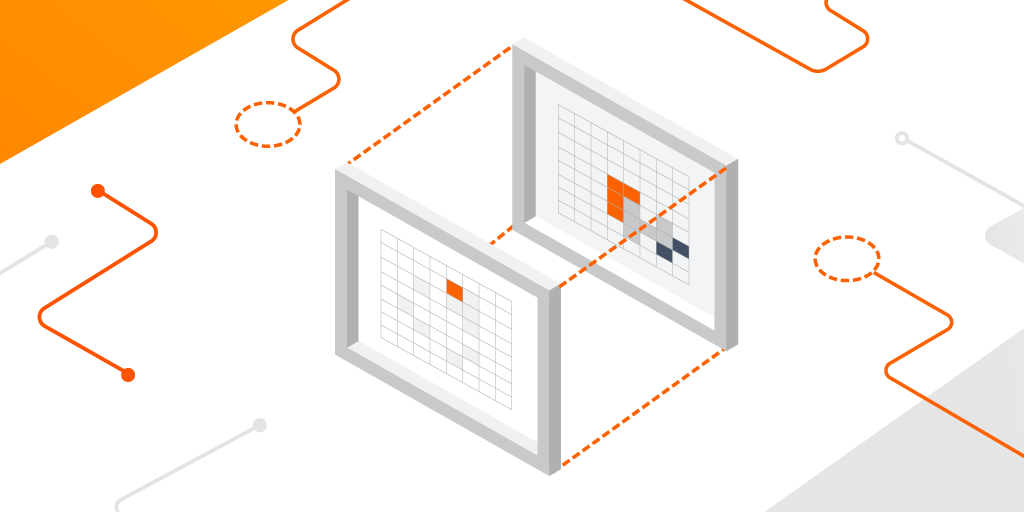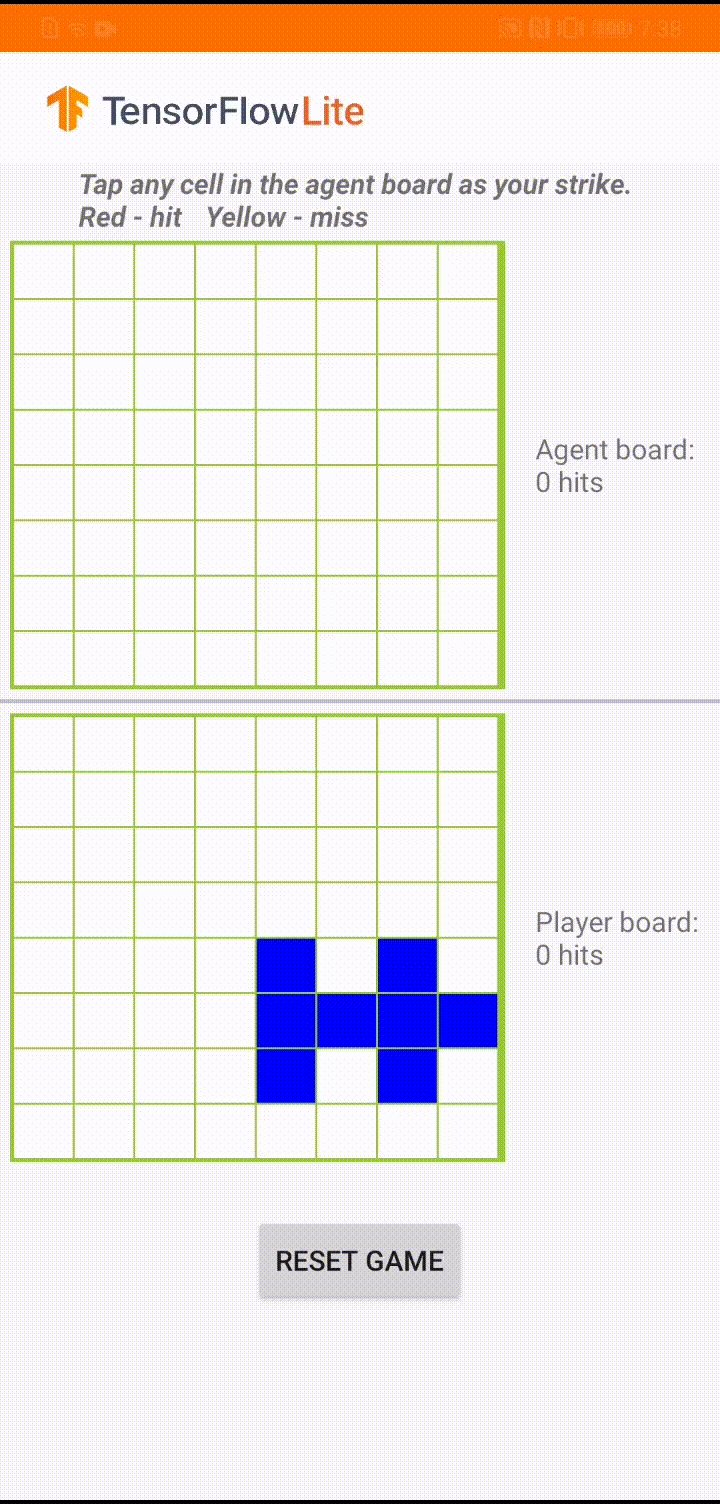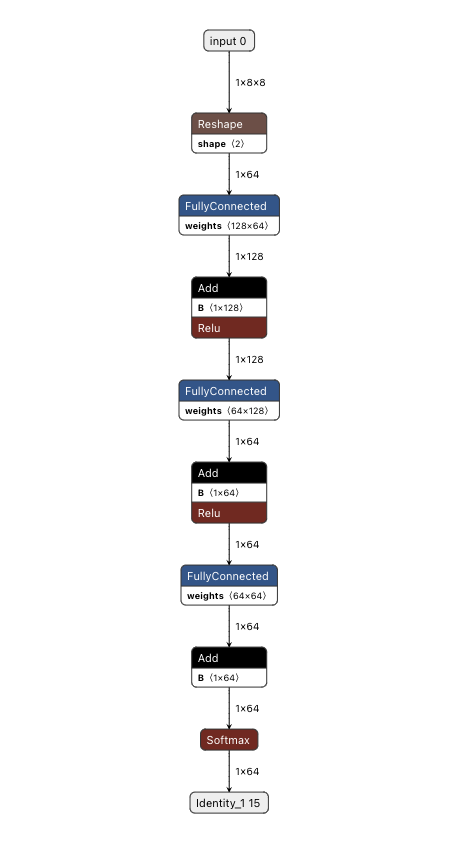Flax · JAX ·Building a reinforcement learning agent with JAX, and deploying it on Android with TensorFlow LitePosted by Wei Wei, Developer Advocate

In our previous blog post Building a board game app with TensorFlow: a new TensorFlow Lite reference app, we showed you how to use TensorFlow and TensorFlow Agents to train a reinforcement learning (RL) agent to play a simple board game ‘Plane Strike’. We also converted the trained model to TensorFlow Lite and then deployed it into a fully-functional Android app. In this blog, we will demonstrate a new path: train the same RL agent with Flax/JAX and deploy it into the same Android app we have built before. The complete code has been open sourced in the tensorflow/examples repository for your reference.

To refresh your memory, our RL-based agent needs to predict a strike position based on the human player’s board position so that it can finish the game before the human player does. For more detailed game rules, please refer to our previous blog.Demo game play in ‘Plane Strike’

### Background: JAX and TensorFlow

JAX is a NumPy-like library developed by Google Research for high performance computing. It uses XLA to compile programs optimized for GPUs and TPUs. Flax is a popular neural network library built on top of JAX. Researchers have been using JAX/Flax to train very large models with billions of parameters (such as PaLM for language understanding and generation, or Imagen for image generation), making full use of modern hardware. If you're new to JAX and Flax, start with this JAX 101 tutorial and this Flax Getting Started example.

TensorFlow started as a library for ML towards the end of 2015 and has since become a rich ecosystem that includes tools for productionizing ML pipelines (TFX), data visualization (TensorBoard), deploying ML models to edge devices (TensorFlow Lite), and devices running on a web browser or any device capable of executing JavaScript (TensorFlow.js). Models developed in JAX or Flax can tap into this rich ecosystem by first converting such a model to the TensorFlow SavedModel format, and then using the same tooling as if they had been developed in TensorFlow natively.

If you already have a JAX-trained model and want to deploy it today, we have put together a list of resources for you:
• This blog post demos how to convert a Flax/JAX model to TFLite and run it in a native Android app
Overall, no matter what your deployment target is (server, web or mobile), we got you covered.
Implementing the game agent with Flax/JAX

Coming back to our board game, to implement our RL agent, we will leverage the same gym environment as before. We will train the same policy gradient model using Flax/JAX this time. Recall that mathematically the policy gradient is defined as:

where:

• T: the number of timesteps per episode, which can vary per episode
• st: the state at timestep t
• at: chosen action at timestep t given state s
• πθ: the policy parameterized by θ
• R(*): the reward gathered, given the policy

We define a 3-layer MLP as our policy network, which predicts the agent’s next strike position.

 class PolicyGradient(nn.Module):  """Neural network to predict the next strike position."""   @nn.compact  def __call__(self, x):    dtype = jnp.float32    x = x.reshape((x.shape, -1))    x = nn.Dense(        features=2 * common.BOARD_SIZE**2, name='hidden1', dtype=dtype)(           x)    x = nn.relu(x)    x = nn.Dense(features=common.BOARD_SIZE**2, name='hidden2', dtype=dtype)(x)    x = nn.relu(x)    x = nn.Dense(features=common.BOARD_SIZE**2, name='logits', dtype=dtype)(x)    policy_probabilities = nn.softmax(x)    return policy_probabilities

In our main training loop, in each iteration we use the neural network to play a round of the game, gather the trajectory information (game board positions, actions taken and rewards), discount the rewards, and then train the model with the trajectories.

 for i in tqdm(range(iterations)):   predict_fn = functools.partial(run_inference, params)   board_log, action_log, result_log = common.play_game(predict_fn)   rewards = common.compute_rewards(result_log)   optimizer, params, opt_state = train_step(optimizer, params, opt_state,                                             board_log, action_log, rewards)

In the train_step() method, we first compute the loss using the trajectories. Then we use jax.grad() to compute the gradients. Lastly we use Optax, a gradient processing and optimization library for JAX, to update the model parameters.

 def compute_loss(logits, labels, rewards):  one_hot_labels = jax.nn.one_hot(labels, num_classes=common.BOARD_SIZE**2)  loss = -jnp.mean(      jnp.sum(one_hot_labels * jnp.log(logits), axis=-1) * jnp.asarray(rewards))  return loss  def train_step(model_optimizer, params, opt_state, game_board_log,              predicted_action_log, action_result_log): """Run one training step."""   def loss_fn(model_params):    logits = run_inference(model_params, game_board_log)    loss = compute_loss(logits, predicted_action_log, action_result_log)    return loss   def compute_grads(params):    return jax.grad(loss_fn)(params)   grads = compute_grads(params)  updates, opt_state = model_optimizer.update(grads, opt_state)  params = optax.apply_updates(params, updates)  return model_optimizer, params, opt_state  @jax.jitdef run_inference(model_params, board):  logits = PolicyGradient().apply({'params': model_params}, board)  return logits

That’s it for the training loop. We can visualize the training progress in TensorBoard as below; here we use the proxy metric ‘game_length’ (the number of steps to finish the game) to track the progress. The intuition is that when the agent becomes smarter, it can finish the game in fewer steps.

### Converting the Flax/JAX model to TensorFlow Lite and integrating with the Android app

After the model is trained, we use the jax2tf, a TensorFlow-JAX interoperation tool, to convert the JAX model into a TensorFlow concrete function. And the final step is to call TensorFlow Lite converter to convert the concrete function into a TFLite model.

 # Convert to tflite model model = PolicyGradient() jax_predict_fn = lambda input: model.apply({'params': params}, input)  tf_predict = tf.function(     jax2tf.convert(jax_predict_fn, enable_xla=False),     input_signature=[         tf.TensorSpec(             shape=[1, common.BOARD_SIZE, common.BOARD_SIZE],             dtype=tf.float32,             name='input')     ],     autograph=False, )  converter = tf.lite.TFLiteConverter.from_concrete_functions(     [tf_predict.get_concrete_function()], tf_predict)  tflite_model = converter.convert()  # Save the model with open(os.path.join(modeldir, 'planestrike.tflite'), 'wb') as f:   f.write(tflite_model)

The JAX-converted TFLite model behaves exactly like any TensorFlow-trained TFLite model. You can visualize it with Netron:Visualizing TFLite model converted from Flax/JAX using Netron
We can use exactly the same Java code as before to invoke the model and get the prediction.

 convertBoardStateToByteBuffer(board);tflite.run(boardData, outputProbArrays);float[] probArray = outputProbArrays;int agentStrikePosition = -1;float maxProb = 0;for (int i = 0; i < probArray.length; i++) {  int x = i / Constants.BOARD_SIZE;  int y = i % Constants.BOARD_SIZE;  if (board[x][y] == BoardCellStatus.UNTRIED && probArray[i] > maxProb) {    agentStrikePosition = i;    maxProb = probArray[i];  }}

### Conclusion

In summary, this article walks you through how to train a simple reinforcement learning model with Flax/JAX, leverage jax2tf to convert it to TensorFlow Lite, and integrate the converted model into an Android app.

Now you have learned how to build neural network models with Flax/JAX, and tap into the powerful TensorFlow ecosystem to deploy your models pretty much anywhere you want. We can’t wait to see the fantastic apps you build with both JAX and TensorFlow!
Next postPosted by Wei Wei, Developer AdvocateIn our previous blog post Building a board game app with TensorFlow: a new TensorFlow Lite reference app, we showed you how to use TensorFlow and TensorFlow Agents to train a reinforcement learning (RL) agent to play a simple board game ‘Plane Strike’. We also converted the trained model to TensorFlow Lite and then deployed it into a fully-functional Android a…